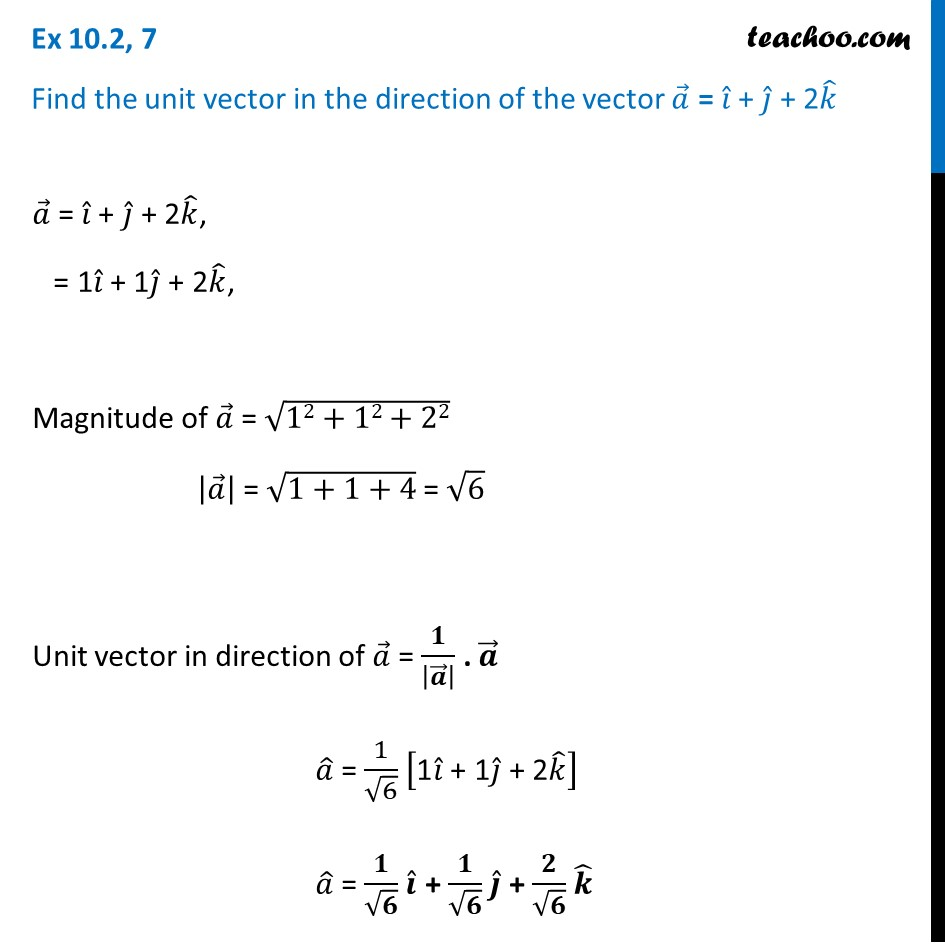Ex 10.2

Chapter 10 Class 12 Vector Algebra
Serial order wiseLearn in your speed, with individual attention - Teachoo Maths 1-on-1 Class

### Transcript

Ex 10.2, 7 Find the unit vector in the direction of the vector 𝑎 ⃗ = 𝑖 ̂ + 𝑗 ̂ + 2𝑘 ̂ 𝑎 ⃗ = 𝑖 ̂ + 𝑗 ̂ + 2𝑘 ̂, = 1𝑖 ̂ + 1𝑗 ̂ + 2𝑘 ̂, Magnitude of 𝑎 ⃗ = √(12+12+22) |𝑎 ⃗ | = √(1+1+4) = √6 Unit vector in direction of 𝑎 ⃗ = 𝟏/|𝒂 ⃗ | . 𝒂 ⃗ 𝑎 ̂ = 1/√6 ["1" 𝑖 ̂" + 1" 𝑗 ̂" + 2" 𝑘 ̂ ] 𝑎 ̂ = 𝟏/√𝟔 𝒊 ̂ + 𝟏/√𝟔 𝒋 ̂ + 𝟐/√𝟔 𝒌 ̂